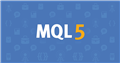# Please double check my function407

By mistake I normalized the values I'm getting from iMA with Digits.

```double MA(int MA_Period, int shift)
{
return(NormalizeDouble(iMA(NULL, 0, MA_Period, 0, 1, 0, shift),Digits));
}```

Then , I was using this for finding the cross. (vice versa for cross down)

``` if(MA(Fast_MA, 1) > MA(Slow_MA, 1) && MA(Fast_MA, 2) < MA(Slow_MA, 2))
```

I found out that it skips some of the crosses because some times in the bar 2, both MAs have the same value. So, the condition above weren't become true.

Before I realize that what cause this problem is that I normalized the value of MAs , I wrote this code to make sure I'm not lose any cross:

```bool MA_Cross_UP()
{
if(MA(Fast_MA, 1) > MA(Slow_MA, 1) && MA(Fast_MA, 2) < MA(Slow_MA, 2))
return true;
if(MA(Fast_MA, 1) > MA(Slow_MA, 1) && MA(Fast_MA, 2) > MA(Slow_MA, 2))
return false;
if(MA(Fast_MA, 1) > MA(Slow_MA, 1))
{
for(int i = 2; i <= 20; i++)
{
if(MA(Fast_MA, i) == MA(Slow_MA, i))
{
continue;
}
if(MA(Fast_MA, i) < MA(Slow_MA, i))
return true;
if(MA(Fast_MA, i) > MA(Slow_MA, i))
return false;
}
}
return false;
}```

My questions are:

1 - Is it possible to have the same value for two MAs if I don't normalize them? In other words do I need to use or is it better to use the function above or I can trust the same simple code?

2- Can you please double check this function and tell me if you see anything wrong with it?

Regards.Documentation on MQL5: Constants, Enumerations and Structures / Named Constants / Predefined Macro Substitutions
• www.mql5.com
//| Expert initialization function                                   | //| Expert deinitialization function                                 | //| Expert tick function                                             | //| test1                                                            |...